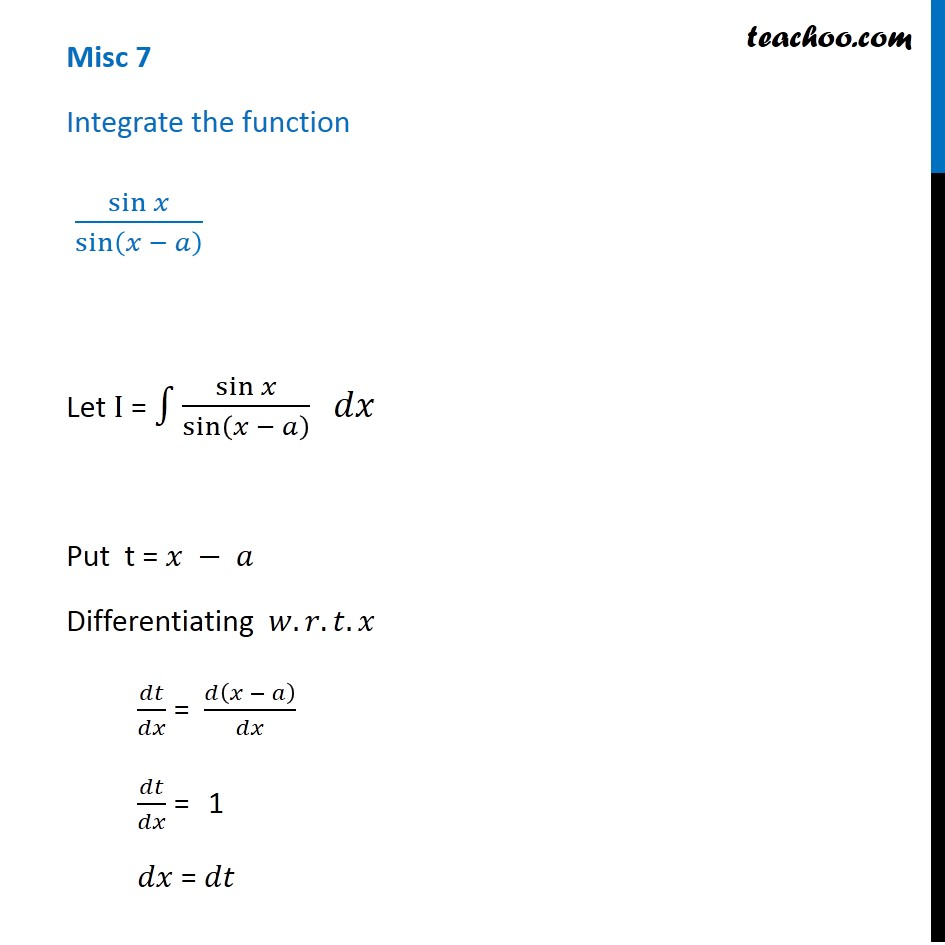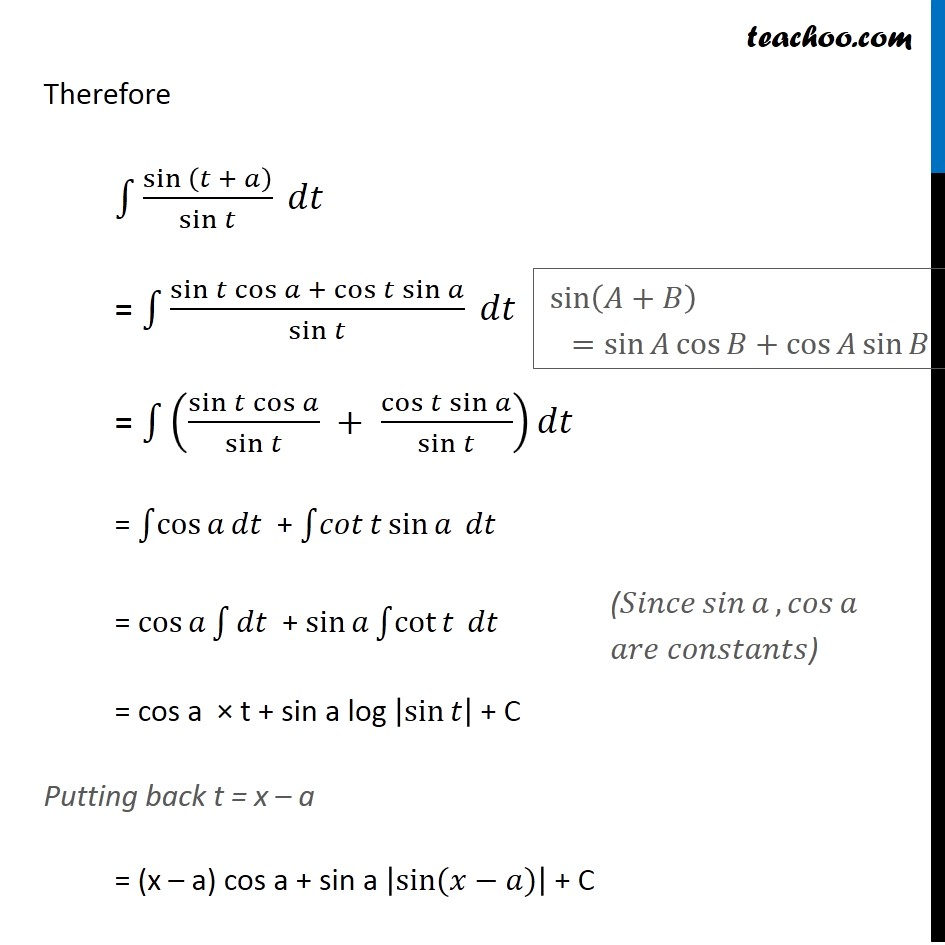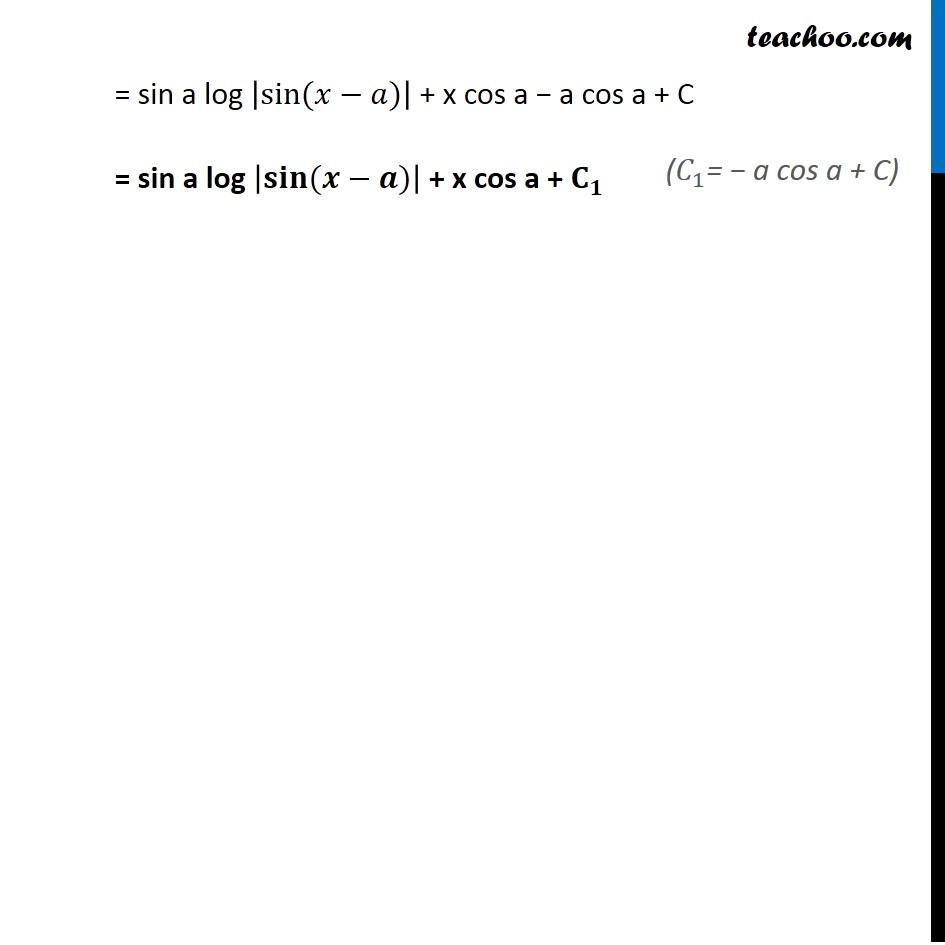Miscellaneous

Chapter 7 Class 12 Integrals
Serial order wiseLearn in your speed, with individual attention - Teachoo Maths 1-on-1 Class

### Transcript

Misc 7 Integrate the function sin⁡𝑥/sin⁡(𝑥 − 𝑎) Let I = ∫1▒sin⁡𝑥/sin⁡(𝑥 − 𝑎) 𝑑𝑥 Put t = 𝑥 − 𝑎 Differentiating 𝑤.𝑟.𝑡.𝑥 𝑑𝑡/𝑑𝑥 = 𝑑(𝑥 − 𝑎)/𝑑𝑥 𝑑𝑡/𝑑𝑥 = 1 𝑑𝑥 = 𝑑𝑡 Therefore ∫1▒〖sin 〗⁡(𝑡 + 𝑎)/sin⁡𝑡 𝑑𝑡 = ∫1▒(sin⁡𝑡 cos⁡𝑎 + cos⁡𝑡 sin⁡𝑎)/sin⁡𝑡 𝑑𝑡 = ∫1▒((sin⁡𝑡 cos⁡𝑎)/sin⁡𝑡 + (cos⁡𝑡 sin⁡𝑎)/sin⁡𝑡 ) 𝑑𝑡 = ∫1▒cos⁡𝑎 𝑑𝑡 + ∫1▒𝑐𝑜𝑡⁡𝑡 sin⁡𝑎 𝑑𝑡 = cos⁡𝑎 ∫1▒𝑑𝑡 + sin⁡𝑎 ∫1▒cot⁡𝑡 𝑑𝑡 = cos a × t + sin a log |sin⁡𝑡 | + C Putting back t = x – a = (x – a) cos a + sin a |sin⁡〖(𝑥−𝑎)〗 | + C sin⁡(𝐴+𝐵) =sin⁡𝐴 cos⁡𝐵+cos⁡𝐴 sin⁡𝐵 (𝑆𝑖𝑛𝑐𝑒 𝑠𝑖𝑛⁡𝑎,𝑐𝑜𝑠⁡𝑎 𝑎𝑟𝑒 𝑐𝑜𝑛𝑠𝑡𝑎𝑛𝑡𝑠) = sin a log |sin⁡〖(𝑥−𝑎)〗 | + x cos a − a cos a + C = sin a log |𝐬𝐢𝐧⁡〖(𝒙−𝒂)〗 | + x cos a + 𝐂_𝟏 (𝐶_1= − a cos a + C)